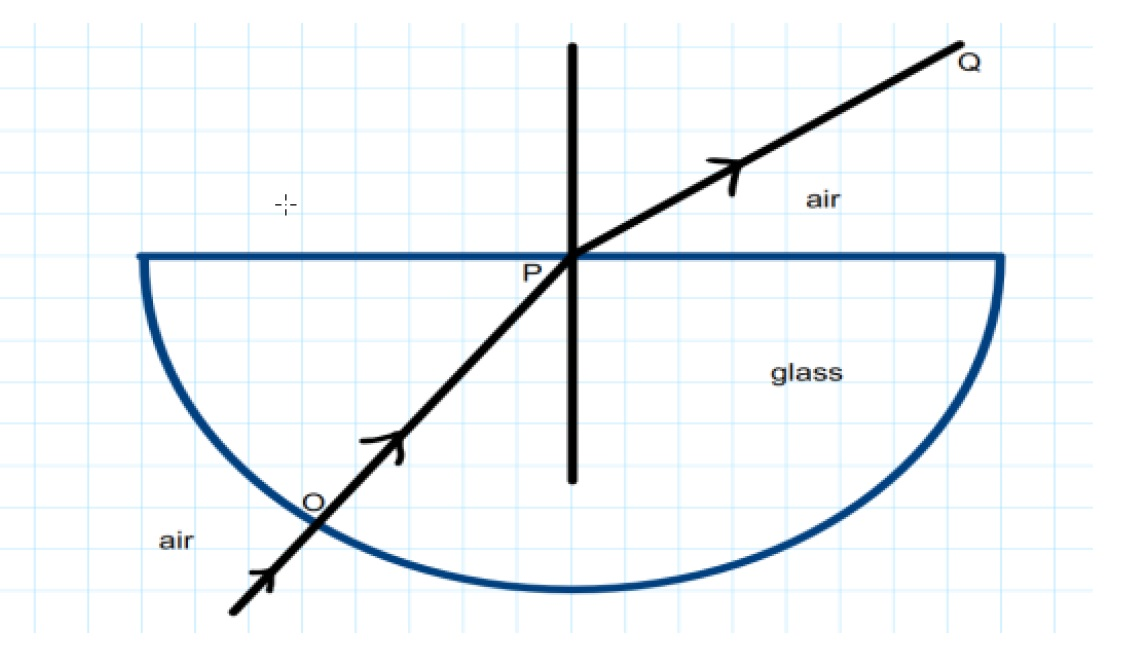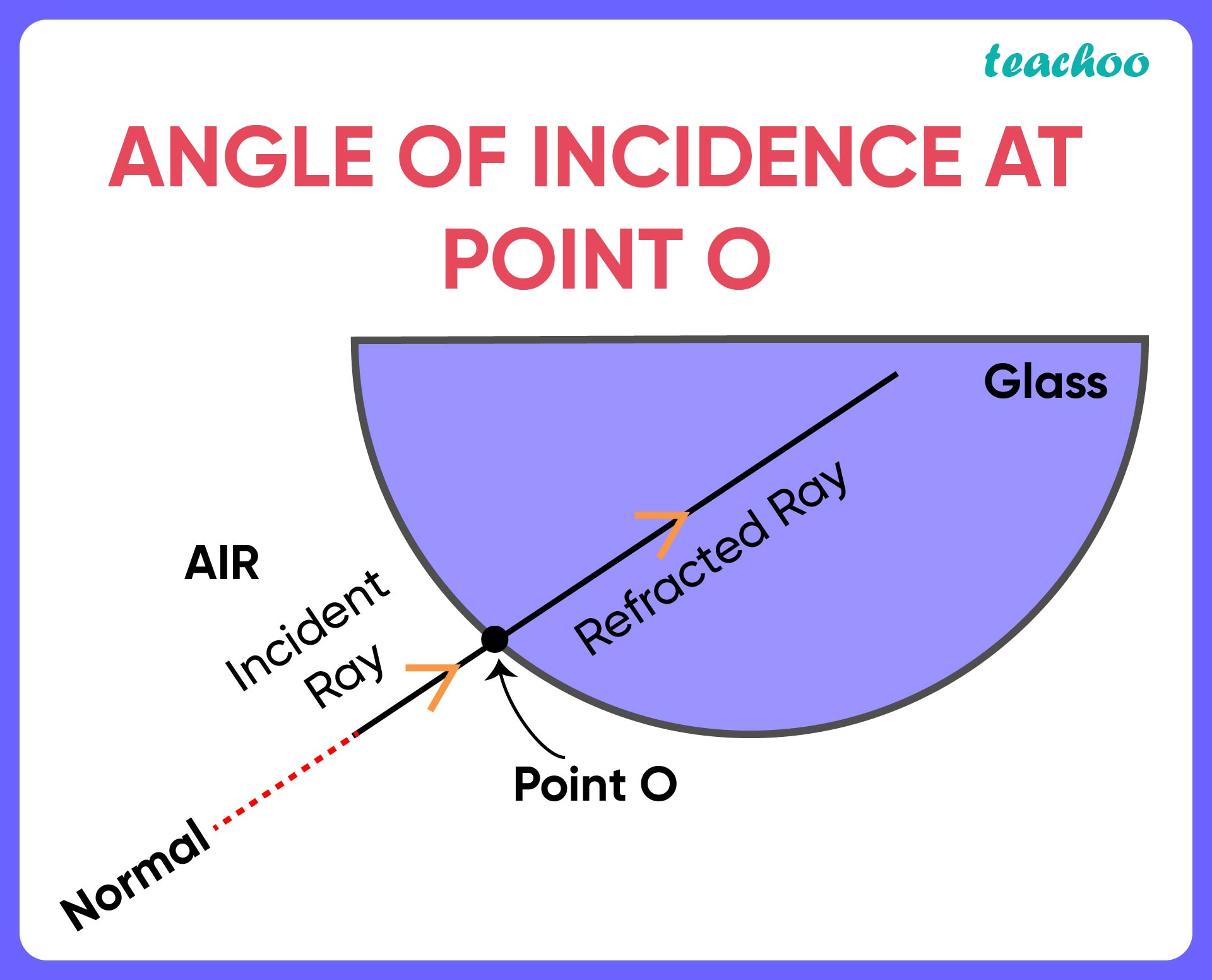## (D) 180°

Angle of incidence is the angle between a incident ray and normal

In our figure, at point OIncident ray and normal are the same line.

Thus, angle of incidence will be 0 degrees

So, the correct answer is (b)

1. Class 10
2. Solutions to CBSE Sample Paper - Science Class 10
3. Solutions - CBSE Class 10 Sample Paper for 2022 Boards - Science [MCQ]

Solutions - CBSE Class 10 Sample Paper for 2022 Boards - Science [MCQ]

Class 10
Solutions to CBSE Sample Paper - Science Class 10
• Solutions - CBSE Class 10 Sample Paper for 2022 Boards - Science [MCQ]